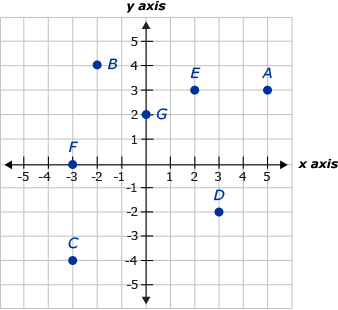# Math

## Introduction

We will begin to graph points in a rectangular coordinate system. Recall that a number line has a point for every real number. The positive numbers are on one side of a point designated as zero and the negative numbers are on the other side of zero. When the number line is horizontal, it is customary to have the positive numbers to the right and the negative numbers to the left of zero. When the number line is vertical, we generally have the positive numbers above and the negative numbers below zero. We use a scale with tick marks to enable us to locate numbers and a darkened dot (point) to graph a specific number.

Because we will be working with equations involving two variables, we will need a system to graph the values for each of these variables and how they relate to each other. This lesson will cover basic graphing where two variables are concerned. We will learn how to graph points in a rectangular coordinate system and determine the location of the points we graph. We will also relate the points we are graphing to equations and their solutions.As a result of this lesson you will be ready to begin graphing linear equations in two variables by creating a table of values. Linear equations can be used in many business applications including depreciation of equipment for income tax deduction purposes and creating cost functions. Many everyday relationships are also linear. You may notice that real data you collect will have a graph that is a line, or at least close to one. Even simple data like your gasoline usage, when comparing miles driven to gallons used, is pretty close to linear. Think about going to a movie with a group of friends. How do you calculate the cost of the evening? The variables are the number of friends and the total cost of the tickets. If a ticket is \$5.00 and one person goes (variable x), then the cost will be y. 5x=y. So, if one person goes, 5(1) =5. If 2 people go, 5(2)=10. There are many more situations in life that involve two sets of numbers that are related to each other. For example, If you know the price of one ticket for a show, you can calculate the cost for any number of people to attend. Similarly, if you know how much gas will cost for one gallon, you can calculate how many gallons you will be able to purchase with the money in your wallet. You may have the following questions as they relate to graphing.

• How do you identify the location of a point?
• How do you plot points with given ordered pairs?
• How do you know if a given ordered pair is a solution of an equation?

## Key Terms

• Axis, axes - A number line. It is used to locate a specific point by using a number. "Axes" is the plural of axis.
• Coordinate - A real number on an axis.
• Coordinates - An ordered set of numbers that define the position of a point.
• Origin - The point of intersection of two axes. It is the point where the value on each axis is zero and is designated by the coordinates (0,0).
• Ordered pair - Used to designate the location of a point in relation to the origin using coordinates which correspond with the numbers on the axes.
• Quadrant - Four regions that are bounded by the axes.
• Solution to an equation in two variables – A solution to an equation in two variables if the numbers in that ordered pair make the equation true when they are substituted into the equation.

## Sample Question

Find the ordered pair of points A, B, C, D, E, F, and G on the graph below.Question 1 of

A

B

C

D

E

F

G

Throughout this lesson, you will learn how to graph ordered pairs.